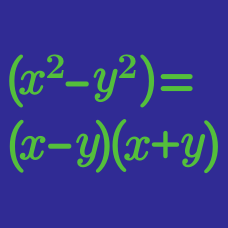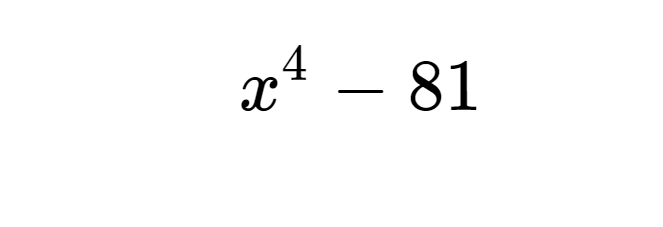Algebra

# Factoring Polynomials Using Identities

Which of the following must be a factor of

$x^3 + 8 ?$

Note: $$a^3 + b^3 = ( a + b ) ( a^2 - ab + b^2 ) .$$

Using the identity $$a^2 - b^2 = (a-b) ( a+b)$$, which of the following must be a factor of

$x^4 + 2500 ?$

If $$a$$ and $$b$$ are positive numbers and $x^4-38x^2y^2+y^4=(x^2+axy-y^2)(x^2-bxy-y^2),$ what is the value of $$a+b$$?

Suppose $$a$$, $$b$$, $$c$$ and $$d$$ are all non-positive integers such that the following is an algebraic identity in $$x$$: $(x-a)^2(x+a)^2=x^4+bx^3+cx^2+dx+256.$ What is the value of $$-a-b-c-d$$?Which of the following is a factor of $$x^4 - 81$$?

×